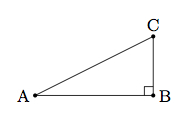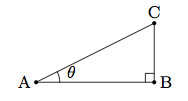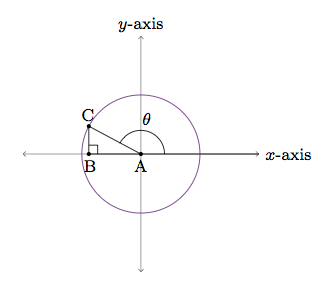# Trigonometric Ratios and the Pythagorean Theorem

Alignments to Content Standards: F-TF.C.81. In the triangle pictured above show that $$\left(\frac{|AB|}{|AC|}\right)^2 + \left(\frac{|BC|}{|AC|}\right)^2 = 1$$
2. Deduce that $\sin^2{\theta} + \cos^2{\theta} = 1$ for any acute angle $\theta$.
3. If $\theta$ is in the second quadrant and $\sin{\theta} = \frac{8}{17}$ what can you say about $\cos{\theta}$? Draw a picture and explain.

## IM Commentary

The purpose of this task is to use the Pythagorean Theorem to establish the fundamental trigonometric identity $\sin^2{\theta} + \cos^2{\theta} = 1$ for an acute angle $\theta$. The reasoning behind this identity is then applied to calculate $\cos{\theta}$ for a given obtuse angle. In order to successfully complete part (c) students must be familiar with the definitions of trigonometric functions for arbitrary angles using the unit circle (F-TF.2).

The Pythagorean Theorem requires $\triangle ABC$ to be a right triangle and so $\angle A$ must be acute. So the reasoning in parts a and b of this task establish the identity $$\sin^2{\theta} + \cos^2{\theta} = 1$$ for acute angles only. Using the unit circle as in part c, however, shows that this identity is true for all angles. More about this can be found in http://www.illustrativemathematics.org/tasks/1692.

## Solution

1. The Pythagorean Theorem says that if $\triangle ABC$ is a right triangle with right angle $B$ then $|AB|^2 + |BC|^2 = |AC|^2$. Dividing both sides by $|AC|^2$ gives $$\left(\frac{|AB|}{|AC|}\right)^2 + \left(\frac{|BC|}{|AC|}\right)^2 = 1.$$

2. If $0 \lt m(\theta) \lt 90$, then we can make a right triangle $ABC$, as pictured in the problem statement, so that $m(\angle BAC) = \theta$:Then from part (a) we have $$\left(\frac{|AB|}{|AC|}\right)^2 + \left(\frac{|BC|}{|AC|}\right)^2 = 1.$$ We also know that $\frac{|AB|}{|AC|} = \cos{\theta}$ and $\frac{|BC|}{|AC|} = \sin{\theta}$ so we have $\sin^2{\theta} + \cos^2{\theta} = 1$.

3. Below is a picture of an angle $\theta$ in the second quadrant with $\sin{\theta} = \frac{8}{17}$:In the picture, the purple circle is the unit circle. The coordinates of $C$ are $(\cos{\theta}, \sin{\theta})$ and since $C$ lies on the unit circle we have $$\sin^2{\theta} + \cos^2{\theta} = 1.$$ Since $\sin{\theta} = \frac{8}{17}$ we can solve for $\cos{\theta}$ and we find $\cos{\theta} = \pm \frac{15}{17}$. Since we are in the second quadrant $\cos{\theta} = - \frac{15}{17}$.